# TreeMap原理实现及常用方法 - 工匠初心

## 一. TreeMap概述

1. TreeMap存储K-V键值对，通过红黑树（R-B tree）实现；
2. TreeMap继承了NavigableMap接口，NavigableMap接口继承了SortedMap接口，可支持一系列的导航定位以及导航操作的方法，当然只是提供了接口，需要TreeMap自己去实现；
3. TreeMap实现了Cloneable接口，可被克隆，实现了Serializable接口，可序列化；
4. TreeMap因为是通过红黑树实现，红黑树结构天然支持排序，默认情况下通过Key值的自然顺序进行排序；

## 二. 红黑树回顾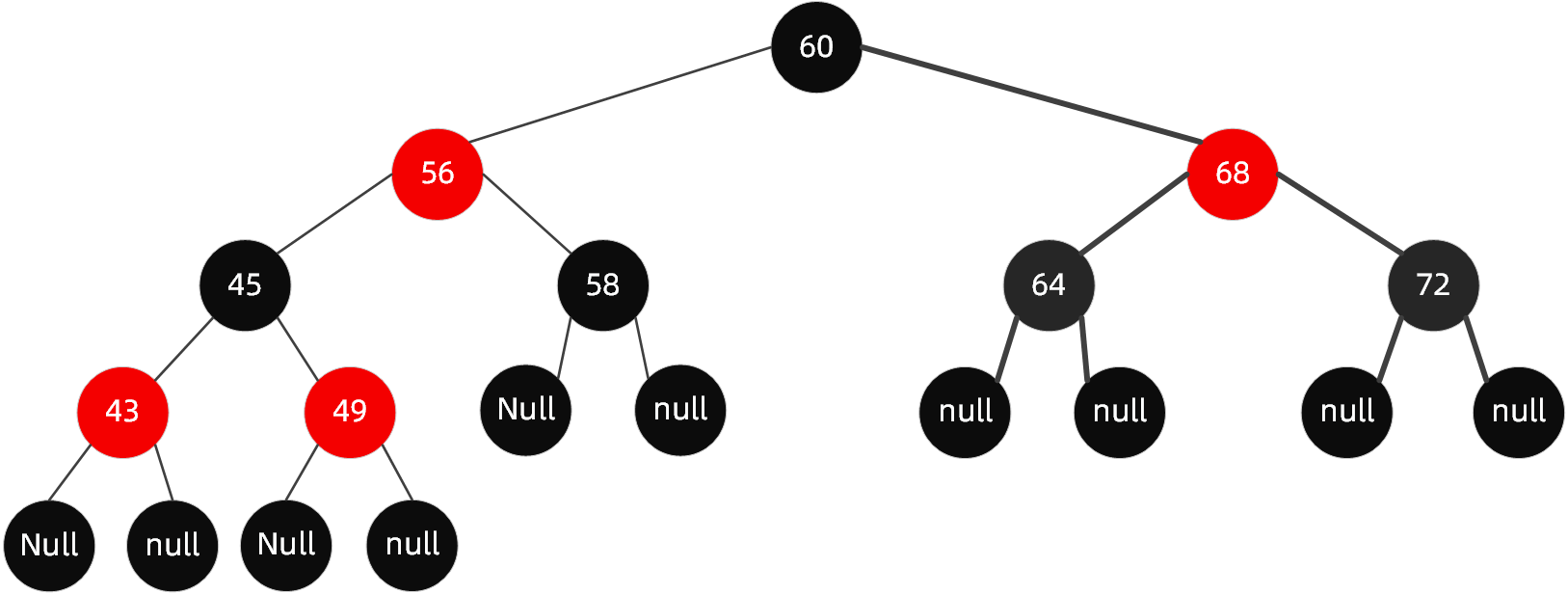1. 节点分为红色或者黑色；
2. 根节点必为黑色；
3. 叶子节点都为黑色，且为null；
4. 连接红色节点的两个子节点都为黑色（红黑树不会出现相邻的红色节点）；
5. 从任意节点出发，到其每个叶子节点的路径中包含相同数量的黑色节点；
6. 新加入到红黑树的节点为红色节点；

1. 变色：在不违反上述红黑树规则特点情况下，将红黑树某个node节点颜色由红变黑，或者由黑变红；
2. 左旋：逆时针旋转两个节点，让一个节点被其右子节点取代，而该节点成为右子节点的左子节点
3. 右旋：顺时针旋转两个节点，让一个节点被其左子节点取代，而该节点成为左子节点的右子节点

## 三. TreeMap构造

``````/**
* 我们前面提到TreeMap是可以自动排序的，默认情况下comparator为null，这个时候按照key的自然顺序进行排
* 序，然而并不是所有情况下都可以直接使用key的自然顺序，有时候我们想让Map的自动排序按照我们自己的规则，
* 这个时候你就需要传递Comparator的实现类
*/
private final Comparator<? super K> comparator;

/**
* TreeMap的存储结构既然是红黑树，那么必然会有唯一的根节点。
*/
private transient Entry<K,V> root;

/**
* Map中key-val对的数量，也即是红黑树中节点Entry的数量
*/
private transient int size = 0;

/**
* 红黑树结构的调整次数
*/
private transient int modCount = 0;
``````

``````static final class Entry<K,V> implements Map.Entry<K,V> {
//key,val是存储的原始数据
K key;
V value;
//定义了节点的左孩子
Entry<K,V> left;
//定义了节点的右孩子
Entry<K,V> right;
//通过该节点可以反过来往上找到自己的父亲
Entry<K,V> parent;
//默认情况下为黑色节点，可调整
boolean color = BLACK;

/**
* 构造器
*/
Entry(K key, V value, Entry<K,V> parent) {
this.key = key;
this.value = value;
this.parent = parent;
}

/**
* 获取节点的key值
*/
public K getKey() {return key;}

/**
* 获取节点的value值
*/
public V getValue() {return value;}

/**
* 用新值替换当前值，并返回当前值
*/
public V setValue(V value) {
V oldValue = this.value;
this.value = value;
return oldValue;
}

public boolean equals(Object o) {
if (!(o instanceof Map.Entry))
return false;
Map.Entry<?,?> e = (Map.Entry<?,?>)o;
return valEquals(key,e.getKey()) && valEquals(value,e.getValue());
}

public int hashCode() {
int keyHash = (key==null ? 0 : key.hashCode());
int valueHash = (value==null ? 0 : value.hashCode());
return keyHash ^ valueHash;
}

public String toString() {
return key + "=" + value;
}
}
``````

Entry静态内部类实现了Map的内部接口Entry，提供了红黑树存储结构的java实现，通过left属性可以建立左子树，通过right属性可以建立右子树，通过parent可以往上找到父节点。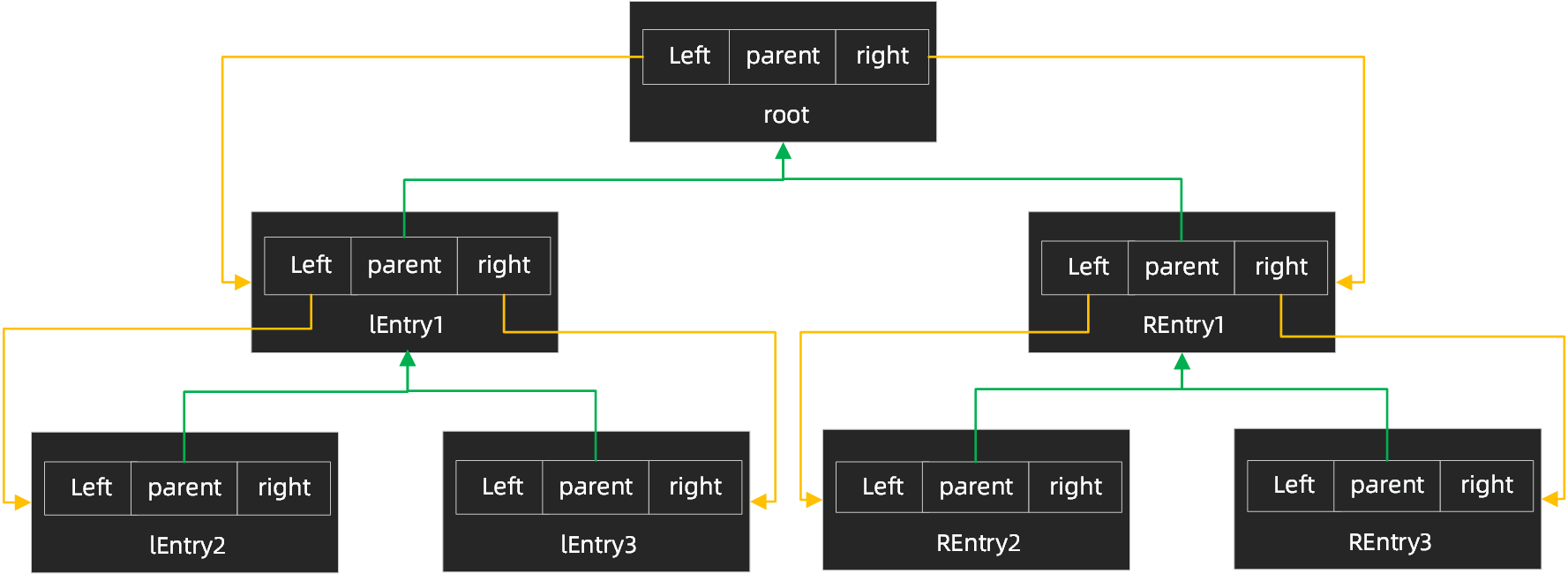TreeMap构造函数：

``````//默认构造函数，按照key的自然顺序排列
public TreeMap() {comparator = null;}
//传递Comparator具体实现，按照该实现规则进行排序
public TreeMap(Comparator<? super K> comparator) {this.comparator = comparator;}
//传递一个map实体构建TreeMap,按照默认规则排序
public TreeMap(Map<? extends K, ? extends V> m) {
comparator = null;
putAll(m);
}
//传递一个map实体构建TreeMap,按照传递的map的排序规则进行排序
public TreeMap(SortedMap<K, ? extends V> m) {
comparator = m.comparator();
try {
buildFromSorted(m.size(), m.entrySet().iterator(), null, null);
} catch (java.io.IOException cannotHappen) {
} catch (ClassNotFoundException cannotHappen) {
}
}
``````

## 四. put方法

put方法为Map的核心方法，TreeMap的put方法大概流程如下：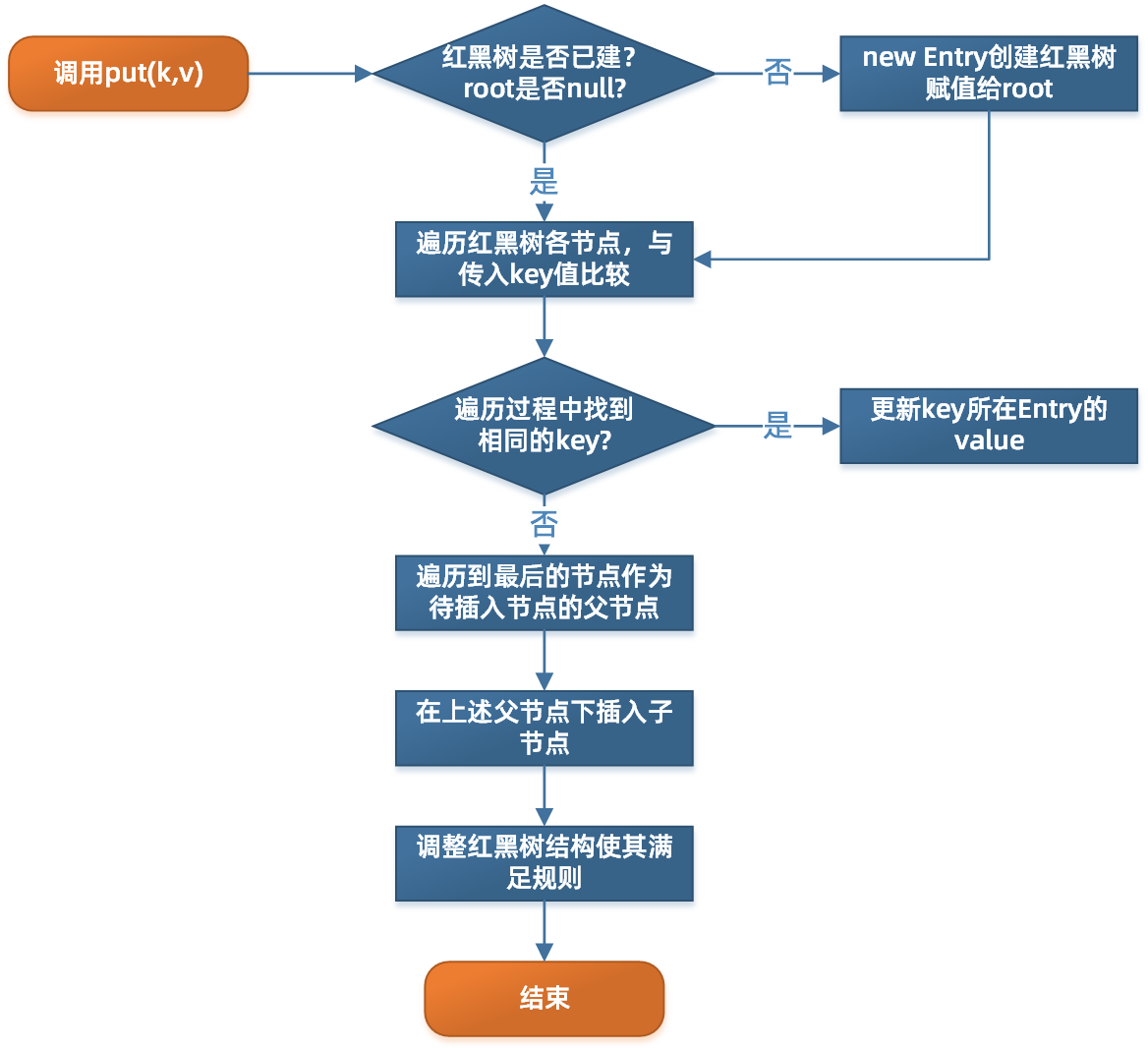``````public V put(K key, V value) {
Entry<K,V> t = root;
/**
* 如果根节点都为null，还没建立起来红黑树，我们先new Entry并赋值给root把红黑树建立起来，这个时候红
* 黑树中已经有一个节点了，同时修改操作+1。
*/
if (t == null) {
compare(key, key);
root = new Entry<>(key, value, null);
size = 1;
modCount++;
return null;
}
/**
* 如果节点不为null,定义一个cmp，这个变量用来进行二分查找时的比较；定义parent，是new Entry时必须
* 要的参数
*/
int cmp;
Entry<K,V> parent;
// cpr表示有无自己定义的排序规则，分两种情况遍历执行
Comparator<? super K> cpr = comparator;
if (cpr != null) {
/**
* 从root节点开始遍历，通过二分查找逐步向下找
* 第一次循环：从根节点开始，这个时候parent就是根节点，然后通过自定义的排序算法
* cpr.compare(key, t.key)比较传入的key和根节点的key值，如果传入的key<root.key，那么
* 继续在root的左子树中找，从root的左孩子节点（root.left）开始：如果传入的key>root.key,
* 那么继续在root的右子树中找，从root的右孩子节点（root.right）开始;如果恰好key==root.key，
* 那么直接根据root节点的value值即可。
* 后面的循环规则一样，当遍历到的当前节点作为起始节点，逐步往下找
*
* 需要注意的是：这里并没有对key是否为null进行判断，建议自己的实现Comparator时应该要考虑在内
*/
do {
parent = t;
cmp = cpr.compare(key, t.key);
if (cmp < 0)
t = t.left;
else if (cmp > 0)
t = t.right;
else
return t.setValue(value);
} while (t != null);
}
else {
//从这里看出，当默认排序时，key值是不能为null的
if (key == null)
throw new NullPointerException();
@SuppressWarnings("unchecked")
Comparable<? super K> k = (Comparable<? super K>) key;
//这里的实现逻辑和上面一样，都是通过二分查找，就不再多说了
do {
parent = t;
cmp = k.compareTo(t.key);
if (cmp < 0)
t = t.left;
else if (cmp > 0)
t = t.right;
else
return t.setValue(value);
} while (t != null);
}
/**
* 能执行到这里，说明前面并没有找到相同的key,节点已经遍历到最后了，我们只需要new一个Entry放到
* parent下面即可，但放到左子节点上还是右子节点上，就需要按照红黑树的规则来。
*/
Entry<K,V> e = new Entry<>(key, value, parent);
if (cmp < 0)
parent.left = e;
else
parent.right = e;
/**
* 节点加进去了，并不算完，我们在前面红黑树原理章节提到过，一般情况下加入节点都会对红黑树的结构造成
* 破坏，我们需要通过一些操作来进行自动平衡处置，如【变色】【左旋】【右旋】
*/
fixAfterInsertion(e);
size++;
modCount++;
return null;
}
``````

put方法源码中通过fixAfterInsertion(e)方法来进行自平衡处理，我们回顾一下插入时自平衡调整的逻辑，下表中看不懂的名词可以参考关于红黑树(R-B tree)原理，看这篇如何

``````private void fixAfterInsertion(Entry<K,V> x) {
//新插入的节点为红色节点
x.color = RED;
//我们知道父节点为黑色时，并不需要进行树结构调整，只有当父节点为红色时，才需要调整
while (x != null && x != root && x.parent.color == RED) {
//如果父节点是左节点，对应上表中情况1和情况2
if (parentOf(x) == leftOf(parentOf(parentOf(x)))) {
Entry<K,V> y = rightOf(parentOf(parentOf(x)));
//如果叔父节点为红色，对应于“父节点和叔父节点都为红色”，此时通过变色即可实现平衡
//此时父节点和叔父节点都设置为黑色，祖父节点设置为红色
if (colorOf(y) == RED) {
setColor(parentOf(x), BLACK);
setColor(y, BLACK);
setColor(parentOf(parentOf(x)), RED);
x = parentOf(parentOf(x));
} else {
//如果插入节点是黑色，插入的是右子节点，通过【左右节点旋转】（这里先进行父节点左旋）
if (x == rightOf(parentOf(x))) {
x = parentOf(x);
rotateLeft(x);
}
//设置父节点和祖父节点颜色
setColor(parentOf(x), BLACK);
setColor(parentOf(parentOf(x)), RED);
//进行祖父节点右旋（这里【变色】和【旋转】并没有严格的先后顺序，达成目的就行）
rotateRight(parentOf(parentOf(x)));
}
} else {
//父节点是右节点的情况
Entry<K,V> y = leftOf(parentOf(parentOf(x)));
//对应于“父节点和叔父节点都为红色”，此时通过变色即可实现平衡
if (colorOf(y) == RED) {
setColor(parentOf(x), BLACK);
setColor(y, BLACK);
setColor(parentOf(parentOf(x)), RED);
x = parentOf(parentOf(x));
} else {
//如果插入节点是黑色，插入的是左子节点，通过【右左节点旋转】（这里先进行父节点右旋）
if (x == leftOf(parentOf(x))) {
x = parentOf(x);
rotateRight(x);
}
setColor(parentOf(x), BLACK);
setColor(parentOf(parentOf(x)), RED);
//进行祖父节点左旋（这里【变色】和【旋转】并没有严格的先后顺序，达成目的就行）
rotateLeft(parentOf(parentOf(x)));
}
}
}
//根节点必须为黑色
root.color = BLACK;
}
``````

``````private void rotateLeft(Entry<K,V> p) {
if (p != null) {
/**
* 断开当前节点p与其右子节点的关联，重新将节点p的右子节点的地址指向节点p的右子节点的左子节点
* 这个时候节点r没有父节点
*/
Entry<K,V> r = p.right;
p.right = r.left;
//将节点p作为节点r的父节点
if (r.left != null)
r.left.parent = p;
//将节点p的父节点和r的父节点指向同一处
r.parent = p.parent;
//p的父节点为null，则将节点r设置为root
if (p.parent == null)
root = r;
//如果节点p是左子节点，则将该左子节点替换为节点r
else if (p.parent.left == p)
p.parent.left = r;
//如果节点p为右子节点，则将该右子节点替换为节点r
else
p.parent.right = r;
//重新建立p与r的关系
r.left = p;
p.parent = r;
}
}
``````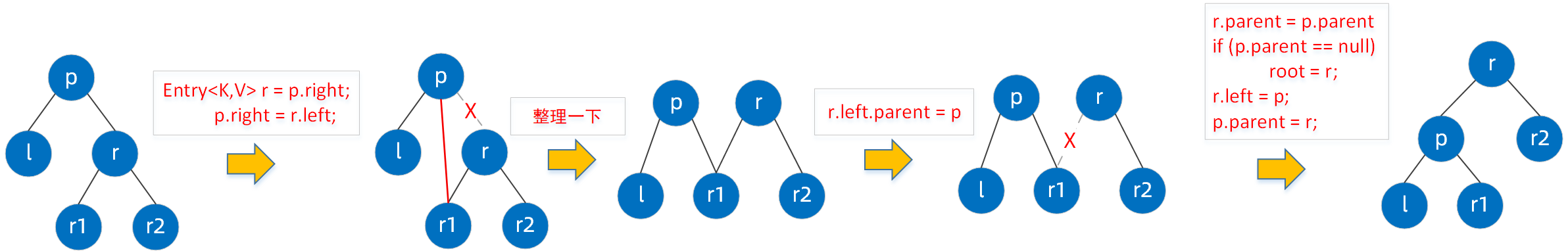## 五. get 方法

get方法是通过二分查找的思想，我们看一下源码

``````public V get(Object key) {
Entry<K,V> p = getEntry(key);
return (p==null ? null : p.value);
}
/**
* 从root节点开始遍历，通过二分查找逐步向下找
* 第一次循环：从根节点开始，这个时候parent就是根节点，然后通过k.compareTo(p.key)比较传入的key和
* 根节点的key值；
* 如果传入的key<root.key, 那么继续在root的左子树中找，从root的左孩子节点（root.left）开始；
* 如果传入的key>root.key, 那么继续在root的右子树中找，从root的右孩子节点（root.right）开始;
* 如果恰好key==root.key，那么直接根据root节点的value值即可。
* 后面的循环规则一样，当遍历到的当前节点作为起始节点，逐步往下找
*/
//默认排序情况下的查找
final Entry<K,V> getEntry(Object key) {

if (comparator != null)
return getEntryUsingComparator(key);
if (key == null)
throw new NullPointerException();
@SuppressWarnings("unchecked")
Comparable<? super K> k = (Comparable<? super K>) key;
Entry<K,V> p = root;
while (p != null) {
int cmp = k.compareTo(p.key);
if (cmp < 0)
p = p.left;
else if (cmp > 0)
p = p.right;
else
return p;
}
return null;
}
/**
* 从root节点开始遍历，通过二分查找逐步向下找
* 第一次循环：从根节点开始，这个时候parent就是根节点，然后通过自定义的排序算法
* cpr.compare(key, t.key)比较传入的key和根节点的key值，如果传入的key<root.key，那么
* 继续在root的左子树中找，从root的左孩子节点（root.left）开始：如果传入的key>root.key,
* 那么继续在root的右子树中找，从root的右孩子节点（root.right）开始;如果恰好key==root.key，
* 那么直接根据root节点的value值即可。
* 后面的循环规则一样，当遍历到的当前节点作为起始节点，逐步往下找
*/
//自定义排序规则下的查找
final Entry<K,V> getEntryUsingComparator(Object key) {
@SuppressWarnings("unchecked")
K k = (K) key;
Comparator<? super K> cpr = comparator;
if (cpr != null) {
Entry<K,V> p = root;
while (p != null) {
int cmp = cpr.compare(k, p.key);
if (cmp < 0)
p = p.left;
else if (cmp > 0)
p = p.right;
else
return p;
}
}
return null;
}
``````

## 六. remove方法

remove方法可以分为两个步骤，先是找到这个节点，直接调用了上面介绍的getEntry(Object key)，这个步骤我们就不说了，直接说第二个步骤，找到后的删除操作。

``````public V remove(Object key) {
Entry<K,V> p = getEntry(key);
if (p == null)
return null;

V oldValue = p.value;
deleteEntry(p);
return oldValue;
}
``````

1. 删除的是根节点，则直接将根节点置为null;
2. 待删除节点的左右子节点都为null，删除时将该节点置为null;
3. 待删除节点的左右子节点有一个有值，则用有值的节点替换该节点即可；
4. 待删除节点的左右子节点都不为null，则找前驱或者后继，将前驱或者后继的值复制到该节点中，然后删除前驱或者后继（前驱：左子树中值最大的节点，后继：右子树中值最小的节点）；
``````private void deleteEntry(Entry<K,V> p) {
modCount++;
size--;
//当左右子节点都不为null时，通过successor(p)遍历红黑树找到前驱或者后继
if (p.left != null && p.right != null) {
Entry<K,V> s = successor(p);
//将前驱或者后继的key和value复制到当前节点p中，然后删除节点s(通过将节点p引用指向s)
p.key = s.key;
p.value = s.value;
p = s;
}
Entry<K,V> replacement = (p.left != null ? p.left : p.right);
/**
* 至少有一个子节点不为null，直接用这个有值的节点替换掉当前节点，给replacement的parent属性赋值,给
* parent节点的left属性和right属性赋值，同时要记住叶子节点必须为null,然后用fixAfterDeletion方法
* 进行自平衡处理
*/
if (replacement != null) {
//将待删除节点的子节点挂到待删除节点的父节点上。
replacement.parent = p.parent;
if (p.parent == null)
root = replacement;
else if (p == p.parent.left)
p.parent.left  = replacement;
else
p.parent.right = replacement;
p.left = p.right = p.parent = null;
/**
* p如果是红色节点的话，那么其子节点replacement必然为红色的，并不影响红黑树的结构
* 但如果p为黑色节点的话，那么其父节点以及子节点都可能是红色的，那么很明显可能会存在红色相连的情
* 况，因此需要进行自平衡的调整
*/
if (p.color == BLACK)
fixAfterDeletion(replacement);
} else if (p.parent == null) {//这种情况就不用多说了吧
root = null;
} else {
/**
* 如果p节点为黑色，那么p节点删除后，就可能违背每个节点到其叶子节点路径上黑色节点数量一致的规则，
* 因此需要进行自平衡的调整
*/
if (p.color == BLACK)
fixAfterDeletion(p);
if (p.parent != null) {
if (p == p.parent.left)
p.parent.left = null;
else if (p == p.parent.right)
p.parent.right = null;
p.parent = null;
}
}
}
``````

``````private void fixAfterDeletion(Entry<K,V> x) {
/**
* 当x不是root节点且颜色为黑色时
*/
while (x != root && colorOf(x) == BLACK) {
/**
* 首先分为两种情况，当前节点x是左节点或者当前节点x是右节点，这两种情况下面都是四种场景,这里通过
* 代码分析一下x为左节点的情况，右节点可参考左节点理解，因为它们非常类似
*/
if (x == leftOf(parentOf(x))) {
Entry<K,V> sib = rightOf(parentOf(x));

/**
* 场景1：当x是左黑色节点，兄弟节点sib是红色节点
* 兄弟节点由红转黑，父节点由黑转红，按父节点左旋，
* 左旋后树的结构变化了，这时重新赋值sib，这个时候sib指向了x的兄弟节点
*/
if (colorOf(sib) == RED) {
setColor(sib, BLACK);
setColor(parentOf(x), RED);
rotateLeft(parentOf(x));
sib = rightOf(parentOf(x));
}

/**
* 场景2：节点x、x的兄弟节点sib、sib的左子节点和右子节点都为黑色时，需要将该节点sib由黑变
* 红，同时将x指向当前x的父节点
*/
if (colorOf(leftOf(sib))  == BLACK &&
colorOf(rightOf(sib)) == BLACK) {
setColor(sib, RED);
x = parentOf(x);
} else {
/**
* 场景3：节点x、x的兄弟节点sib、sib的右子节点都为黑色，sib的左子节点为红色时，
* 需要将sib左子节点设置为黑色，sib节点设置为红色，同时按sib右旋，再将sib指向x的
* 兄弟节点
*/
if (colorOf(rightOf(sib)) == BLACK) {
setColor(leftOf(sib), BLACK);
setColor(sib, RED);
rotateRight(sib);
sib = rightOf(parentOf(x));
}
/**
* 场景4：节点x、x的兄弟节点sib都为黑色，而sib的左右子节点都为红色或者右子节点为红色、
* 左子节点为黑色，此时需要将sib节点的颜色设置成和x的父节点p相同的颜色，
* 设置x的父节点为黑色，设置sib右子节点为黑色，左旋x的父节点p，然后将x赋值为root
*/
setColor(sib, colorOf(parentOf(x)));
setColor(parentOf(x), BLACK);
setColor(rightOf(sib), BLACK);
rotateLeft(parentOf(x));
x = root;
}
} else {//x是右节点的情况
Entry<K,V> sib = leftOf(parentOf(x));

if (colorOf(sib) == RED) {
setColor(sib, BLACK);
setColor(parentOf(x), RED);
rotateRight(parentOf(x));
sib = leftOf(parentOf(x));
}

if (colorOf(rightOf(sib)) == BLACK &&
colorOf(leftOf(sib)) == BLACK) {
setColor(sib, RED);
x = parentOf(x);
} else {
if (colorOf(leftOf(sib)) == BLACK) {
setColor(rightOf(sib), BLACK);
setColor(sib, RED);
rotateLeft(sib);
sib = leftOf(parentOf(x));
}
setColor(sib, colorOf(parentOf(x)));
setColor(parentOf(x), BLACK);
setColor(leftOf(sib), BLACK);
rotateRight(parentOf(x));
x = root;
}
}
}

setColor(x, BLACK);
}
``````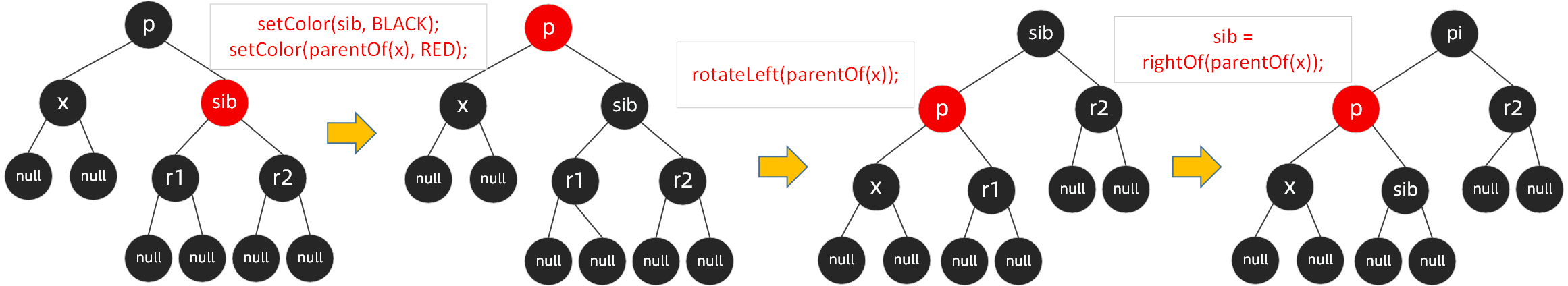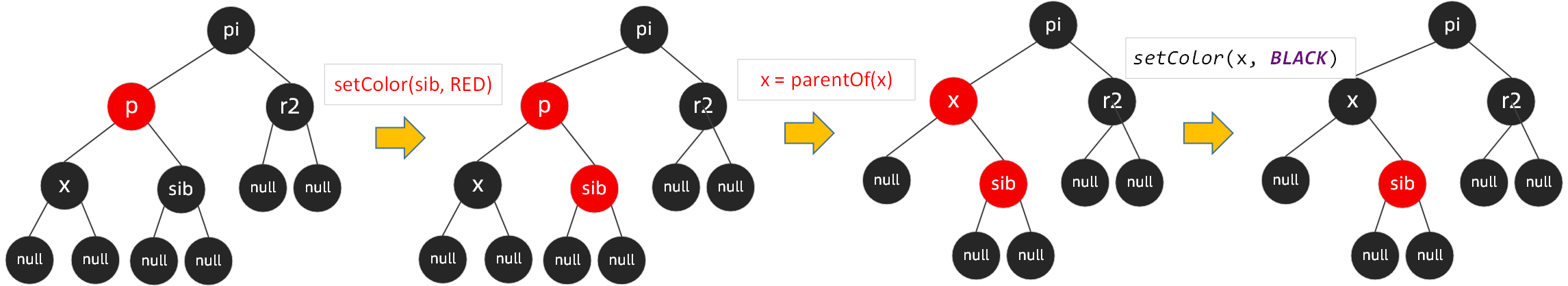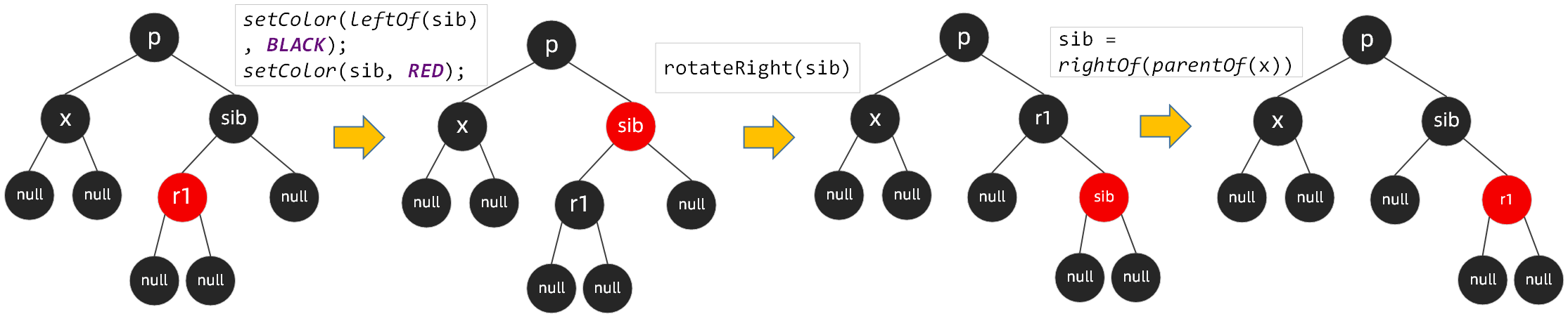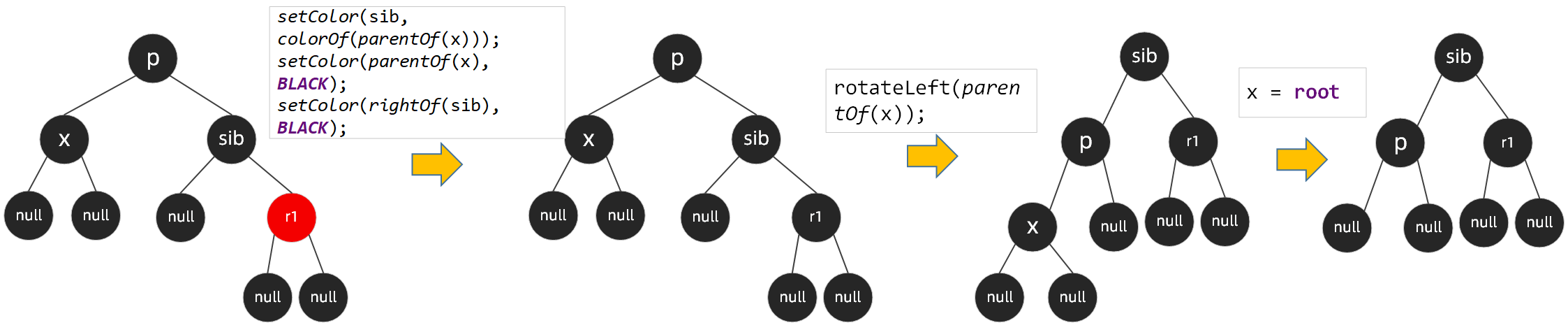## 八. 总结

0 回复

• 请尽量让自己的回复能够对别人有帮助
• 支持 Markdown 格式, **粗体**、~~删除线~~、``单行代码``
• 支持 @ 本站用户；支持表情（输入 : 提示），见 Emoji cheat sheet
• 图片支持拖拽、截图粘贴等方式上传

## 一. TreeMap概述

1. TreeMap存储K-V键值对，通过红黑树（R-B tree）实现；
2. TreeMap继承了NavigableMap接口，NavigableMap接口继承了SortedMap接口，可支持一系列的导航定位以及导航操作的方法，当然只是提供了接口，需要TreeMap自己去实现；
3. TreeMap实现了Cloneable接口，可被克隆，实现了Serializable接口，可序列化；
4. TreeMap因为是通过红黑树实现，红黑树结构天然支持排序，默认情况下通过Key值的自然顺序进行排序；

## 二. 红黑树回顾1. 节点分为红色或者黑色；
2. 根节点必为黑色；
3. 叶子节点都为黑色，且为null；
4. 连接红色节点的两个子节点都为黑色（红黑树不会出现相邻的红色节点）；
5. 从任意节点出发，到其每个叶子节点的路径中包含相同数量的黑色节点；
6. 新加入到红黑树的节点为红色节点；

1. 变色：在不违反上述红黑树规则特点情况下，将红黑树某个node节点颜色由红变黑，或者由黑变红；
2. 左旋：逆时针旋转两个节点，让一个节点被其右子节点取代，而该节点成为右子节点的左子节点
3. 右旋：顺时针旋转两个节点，让一个节点被其左子节点取代，而该节点成为左子节点的右子节点

## 三. TreeMap构造

``````/**
* 我们前面提到TreeMap是可以自动排序的，默认情况下comparator为null，这个时候按照key的自然顺序进行排
* 序，然而并不是所有情况下都可以直接使用key的自然顺序，有时候我们想让Map的自动排序按照我们自己的规则，
* 这个时候你就需要传递Comparator的实现类
*/
private final Comparator<? super K> comparator;

/**
* TreeMap的存储结构既然是红黑树，那么必然会有唯一的根节点。
*/
private transient Entry<K,V> root;

/**
* Map中key-val对的数量，也即是红黑树中节点Entry的数量
*/
private transient int size = 0;

/**
* 红黑树结构的调整次数
*/
private transient int modCount = 0;
``````

``````static final class Entry<K,V> implements Map.Entry<K,V> {
//key,val是存储的原始数据
K key;
V value;
//定义了节点的左孩子
Entry<K,V> left;
//定义了节点的右孩子
Entry<K,V> right;
//通过该节点可以反过来往上找到自己的父亲
Entry<K,V> parent;
//默认情况下为黑色节点，可调整
boolean color = BLACK;

/**
* 构造器
*/
Entry(K key, V value, Entry<K,V> parent) {
this.key = key;
this.value = value;
this.parent = parent;
}

/**
* 获取节点的key值
*/
public K getKey() {return key;}

/**
* 获取节点的value值
*/
public V getValue() {return value;}

/**
* 用新值替换当前值，并返回当前值
*/
public V setValue(V value) {
V oldValue = this.value;
this.value = value;
return oldValue;
}

public boolean equals(Object o) {
if (!(o instanceof Map.Entry))
return false;
Map.Entry<?,?> e = (Map.Entry<?,?>)o;
return valEquals(key,e.getKey()) && valEquals(value,e.getValue());
}

public int hashCode() {
int keyHash = (key==null ? 0 : key.hashCode());
int valueHash = (value==null ? 0 : value.hashCode());
return keyHash ^ valueHash;
}

public String toString() {
return key + "=" + value;
}
}
``````

Entry静态内部类实现了Map的内部接口Entry，提供了红黑树存储结构的java实现，通过left属性可以建立左子树，通过right属性可以建立右子树，通过parent可以往上找到父节点。TreeMap构造函数：

``````//默认构造函数，按照key的自然顺序排列
public TreeMap() {comparator = null;}
//传递Comparator具体实现，按照该实现规则进行排序
public TreeMap(Comparator<? super K> comparator) {this.comparator = comparator;}
//传递一个map实体构建TreeMap,按照默认规则排序
public TreeMap(Map<? extends K, ? extends V> m) {
comparator = null;
putAll(m);
}
//传递一个map实体构建TreeMap,按照传递的map的排序规则进行排序
public TreeMap(SortedMap<K, ? extends V> m) {
comparator = m.comparator();
try {
buildFromSorted(m.size(), m.entrySet().iterator(), null, null);
} catch (java.io.IOException cannotHappen) {
} catch (ClassNotFoundException cannotHappen) {
}
}
``````

## 四. put方法

put方法为Map的核心方法，TreeMap的put方法大概流程如下：``````public V put(K key, V value) {
Entry<K,V> t = root;
/**
* 如果根节点都为null，还没建立起来红黑树，我们先new Entry并赋值给root把红黑树建立起来，这个时候红
* 黑树中已经有一个节点了，同时修改操作+1。
*/
if (t == null) {
compare(key, key);
root = new Entry<>(key, value, null);
size = 1;
modCount++;
return null;
}
/**
* 如果节点不为null,定义一个cmp，这个变量用来进行二分查找时的比较；定义parent，是new Entry时必须
* 要的参数
*/
int cmp;
Entry<K,V> parent;
// cpr表示有无自己定义的排序规则，分两种情况遍历执行
Comparator<? super K> cpr = comparator;
if (cpr != null) {
/**
* 从root节点开始遍历，通过二分查找逐步向下找
* 第一次循环：从根节点开始，这个时候parent就是根节点，然后通过自定义的排序算法
* cpr.compare(key, t.key)比较传入的key和根节点的key值，如果传入的key<root.key，那么
* 继续在root的左子树中找，从root的左孩子节点（root.left）开始：如果传入的key>root.key,
* 那么继续在root的右子树中找，从root的右孩子节点（root.right）开始;如果恰好key==root.key，
* 那么直接根据root节点的value值即可。
* 后面的循环规则一样，当遍历到的当前节点作为起始节点，逐步往下找
*
* 需要注意的是：这里并没有对key是否为null进行判断，建议自己的实现Comparator时应该要考虑在内
*/
do {
parent = t;
cmp = cpr.compare(key, t.key);
if (cmp < 0)
t = t.left;
else if (cmp > 0)
t = t.right;
else
return t.setValue(value);
} while (t != null);
}
else {
//从这里看出，当默认排序时，key值是不能为null的
if (key == null)
throw new NullPointerException();
@SuppressWarnings("unchecked")
Comparable<? super K> k = (Comparable<? super K>) key;
//这里的实现逻辑和上面一样，都是通过二分查找，就不再多说了
do {
parent = t;
cmp = k.compareTo(t.key);
if (cmp < 0)
t = t.left;
else if (cmp > 0)
t = t.right;
else
return t.setValue(value);
} while (t != null);
}
/**
* 能执行到这里，说明前面并没有找到相同的key,节点已经遍历到最后了，我们只需要new一个Entry放到
* parent下面即可，但放到左子节点上还是右子节点上，就需要按照红黑树的规则来。
*/
Entry<K,V> e = new Entry<>(key, value, parent);
if (cmp < 0)
parent.left = e;
else
parent.right = e;
/**
* 节点加进去了，并不算完，我们在前面红黑树原理章节提到过，一般情况下加入节点都会对红黑树的结构造成
* 破坏，我们需要通过一些操作来进行自动平衡处置，如【变色】【左旋】【右旋】
*/
fixAfterInsertion(e);
size++;
modCount++;
return null;
}
``````

put方法源码中通过fixAfterInsertion(e)方法来进行自平衡处理，我们回顾一下插入时自平衡调整的逻辑，下表中看不懂的名词可以参考关于红黑树(R-B tree)原理，看这篇如何

``````private void fixAfterInsertion(Entry<K,V> x) {
//新插入的节点为红色节点
x.color = RED;
//我们知道父节点为黑色时，并不需要进行树结构调整，只有当父节点为红色时，才需要调整
while (x != null && x != root && x.parent.color == RED) {
//如果父节点是左节点，对应上表中情况1和情况2
if (parentOf(x) == leftOf(parentOf(parentOf(x)))) {
Entry<K,V> y = rightOf(parentOf(parentOf(x)));
//如果叔父节点为红色，对应于“父节点和叔父节点都为红色”，此时通过变色即可实现平衡
//此时父节点和叔父节点都设置为黑色，祖父节点设置为红色
if (colorOf(y) == RED) {
setColor(parentOf(x), BLACK);
setColor(y, BLACK);
setColor(parentOf(parentOf(x)), RED);
x = parentOf(parentOf(x));
} else {
//如果插入节点是黑色，插入的是右子节点，通过【左右节点旋转】（这里先进行父节点左旋）
if (x == rightOf(parentOf(x))) {
x = parentOf(x);
rotateLeft(x);
}
//设置父节点和祖父节点颜色
setColor(parentOf(x), BLACK);
setColor(parentOf(parentOf(x)), RED);
//进行祖父节点右旋（这里【变色】和【旋转】并没有严格的先后顺序，达成目的就行）
rotateRight(parentOf(parentOf(x)));
}
} else {
//父节点是右节点的情况
Entry<K,V> y = leftOf(parentOf(parentOf(x)));
//对应于“父节点和叔父节点都为红色”，此时通过变色即可实现平衡
if (colorOf(y) == RED) {
setColor(parentOf(x), BLACK);
setColor(y, BLACK);
setColor(parentOf(parentOf(x)), RED);
x = parentOf(parentOf(x));
} else {
//如果插入节点是黑色，插入的是左子节点，通过【右左节点旋转】（这里先进行父节点右旋）
if (x == leftOf(parentOf(x))) {
x = parentOf(x);
rotateRight(x);
}
setColor(parentOf(x), BLACK);
setColor(parentOf(parentOf(x)), RED);
//进行祖父节点左旋（这里【变色】和【旋转】并没有严格的先后顺序，达成目的就行）
rotateLeft(parentOf(parentOf(x)));
}
}
}
//根节点必须为黑色
root.color = BLACK;
}
``````

``````private void rotateLeft(Entry<K,V> p) {
if (p != null) {
/**
* 断开当前节点p与其右子节点的关联，重新将节点p的右子节点的地址指向节点p的右子节点的左子节点
* 这个时候节点r没有父节点
*/
Entry<K,V> r = p.right;
p.right = r.left;
//将节点p作为节点r的父节点
if (r.left != null)
r.left.parent = p;
//将节点p的父节点和r的父节点指向同一处
r.parent = p.parent;
//p的父节点为null，则将节点r设置为root
if (p.parent == null)
root = r;
//如果节点p是左子节点，则将该左子节点替换为节点r
else if (p.parent.left == p)
p.parent.left = r;
//如果节点p为右子节点，则将该右子节点替换为节点r
else
p.parent.right = r;
//重新建立p与r的关系
r.left = p;
p.parent = r;
}
}
``````## 五. get 方法

get方法是通过二分查找的思想，我们看一下源码

``````public V get(Object key) {
Entry<K,V> p = getEntry(key);
return (p==null ? null : p.value);
}
/**
* 从root节点开始遍历，通过二分查找逐步向下找
* 第一次循环：从根节点开始，这个时候parent就是根节点，然后通过k.compareTo(p.key)比较传入的key和
* 根节点的key值；
* 如果传入的key<root.key, 那么继续在root的左子树中找，从root的左孩子节点（root.left）开始；
* 如果传入的key>root.key, 那么继续在root的右子树中找，从root的右孩子节点（root.right）开始;
* 如果恰好key==root.key，那么直接根据root节点的value值即可。
* 后面的循环规则一样，当遍历到的当前节点作为起始节点，逐步往下找
*/
//默认排序情况下的查找
final Entry<K,V> getEntry(Object key) {

if (comparator != null)
return getEntryUsingComparator(key);
if (key == null)
throw new NullPointerException();
@SuppressWarnings("unchecked")
Comparable<? super K> k = (Comparable<? super K>) key;
Entry<K,V> p = root;
while (p != null) {
int cmp = k.compareTo(p.key);
if (cmp < 0)
p = p.left;
else if (cmp > 0)
p = p.right;
else
return p;
}
return null;
}
/**
* 从root节点开始遍历，通过二分查找逐步向下找
* 第一次循环：从根节点开始，这个时候parent就是根节点，然后通过自定义的排序算法
* cpr.compare(key, t.key)比较传入的key和根节点的key值，如果传入的key<root.key，那么
* 继续在root的左子树中找，从root的左孩子节点（root.left）开始：如果传入的key>root.key,
* 那么继续在root的右子树中找，从root的右孩子节点（root.right）开始;如果恰好key==root.key，
* 那么直接根据root节点的value值即可。
* 后面的循环规则一样，当遍历到的当前节点作为起始节点，逐步往下找
*/
//自定义排序规则下的查找
final Entry<K,V> getEntryUsingComparator(Object key) {
@SuppressWarnings("unchecked")
K k = (K) key;
Comparator<? super K> cpr = comparator;
if (cpr != null) {
Entry<K,V> p = root;
while (p != null) {
int cmp = cpr.compare(k, p.key);
if (cmp < 0)
p = p.left;
else if (cmp > 0)
p = p.right;
else
return p;
}
}
return null;
}
``````

## 六. remove方法

remove方法可以分为两个步骤，先是找到这个节点，直接调用了上面介绍的getEntry(Object key)，这个步骤我们就不说了，直接说第二个步骤，找到后的删除操作。

``````public V remove(Object key) {
Entry<K,V> p = getEntry(key);
if (p == null)
return null;

V oldValue = p.value;
deleteEntry(p);
return oldValue;
}
``````

1. 删除的是根节点，则直接将根节点置为null;
2. 待删除节点的左右子节点都为null，删除时将该节点置为null;
3. 待删除节点的左右子节点有一个有值，则用有值的节点替换该节点即可；
4. 待删除节点的左右子节点都不为null，则找前驱或者后继，将前驱或者后继的值复制到该节点中，然后删除前驱或者后继（前驱：左子树中值最大的节点，后继：右子树中值最小的节点）；
``````private void deleteEntry(Entry<K,V> p) {
modCount++;
size--;
//当左右子节点都不为null时，通过successor(p)遍历红黑树找到前驱或者后继
if (p.left != null && p.right != null) {
Entry<K,V> s = successor(p);
//将前驱或者后继的key和value复制到当前节点p中，然后删除节点s(通过将节点p引用指向s)
p.key = s.key;
p.value = s.value;
p = s;
}
Entry<K,V> replacement = (p.left != null ? p.left : p.right);
/**
* 至少有一个子节点不为null，直接用这个有值的节点替换掉当前节点，给replacement的parent属性赋值,给
* parent节点的left属性和right属性赋值，同时要记住叶子节点必须为null,然后用fixAfterDeletion方法
* 进行自平衡处理
*/
if (replacement != null) {
//将待删除节点的子节点挂到待删除节点的父节点上。
replacement.parent = p.parent;
if (p.parent == null)
root = replacement;
else if (p == p.parent.left)
p.parent.left  = replacement;
else
p.parent.right = replacement;
p.left = p.right = p.parent = null;
/**
* p如果是红色节点的话，那么其子节点replacement必然为红色的，并不影响红黑树的结构
* 但如果p为黑色节点的话，那么其父节点以及子节点都可能是红色的，那么很明显可能会存在红色相连的情
* 况，因此需要进行自平衡的调整
*/
if (p.color == BLACK)
fixAfterDeletion(replacement);
} else if (p.parent == null) {//这种情况就不用多说了吧
root = null;
} else {
/**
* 如果p节点为黑色，那么p节点删除后，就可能违背每个节点到其叶子节点路径上黑色节点数量一致的规则，
* 因此需要进行自平衡的调整
*/
if (p.color == BLACK)
fixAfterDeletion(p);
if (p.parent != null) {
if (p == p.parent.left)
p.parent.left = null;
else if (p == p.parent.right)
p.parent.right = null;
p.parent = null;
}
}
}
``````

``````private void fixAfterDeletion(Entry<K,V> x) {
/**
* 当x不是root节点且颜色为黑色时
*/
while (x != root && colorOf(x) == BLACK) {
/**
* 首先分为两种情况，当前节点x是左节点或者当前节点x是右节点，这两种情况下面都是四种场景,这里通过
* 代码分析一下x为左节点的情况，右节点可参考左节点理解，因为它们非常类似
*/
if (x == leftOf(parentOf(x))) {
Entry<K,V> sib = rightOf(parentOf(x));

/**
* 场景1：当x是左黑色节点，兄弟节点sib是红色节点
* 兄弟节点由红转黑，父节点由黑转红，按父节点左旋，
* 左旋后树的结构变化了，这时重新赋值sib，这个时候sib指向了x的兄弟节点
*/
if (colorOf(sib) == RED) {
setColor(sib, BLACK);
setColor(parentOf(x), RED);
rotateLeft(parentOf(x));
sib = rightOf(parentOf(x));
}

/**
* 场景2：节点x、x的兄弟节点sib、sib的左子节点和右子节点都为黑色时，需要将该节点sib由黑变
* 红，同时将x指向当前x的父节点
*/
if (colorOf(leftOf(sib))  == BLACK &&
colorOf(rightOf(sib)) == BLACK) {
setColor(sib, RED);
x = parentOf(x);
} else {
/**
* 场景3：节点x、x的兄弟节点sib、sib的右子节点都为黑色，sib的左子节点为红色时，
* 需要将sib左子节点设置为黑色，sib节点设置为红色，同时按sib右旋，再将sib指向x的
* 兄弟节点
*/
if (colorOf(rightOf(sib)) == BLACK) {
setColor(leftOf(sib), BLACK);
setColor(sib, RED);
rotateRight(sib);
sib = rightOf(parentOf(x));
}
/**
* 场景4：节点x、x的兄弟节点sib都为黑色，而sib的左右子节点都为红色或者右子节点为红色、
* 左子节点为黑色，此时需要将sib节点的颜色设置成和x的父节点p相同的颜色，
* 设置x的父节点为黑色，设置sib右子节点为黑色，左旋x的父节点p，然后将x赋值为root
*/
setColor(sib, colorOf(parentOf(x)));
setColor(parentOf(x), BLACK);
setColor(rightOf(sib), BLACK);
rotateLeft(parentOf(x));
x = root;
}
} else {//x是右节点的情况
Entry<K,V> sib = leftOf(parentOf(x));

if (colorOf(sib) == RED) {
setColor(sib, BLACK);
setColor(parentOf(x), RED);
rotateRight(parentOf(x));
sib = leftOf(parentOf(x));
}

if (colorOf(rightOf(sib)) == BLACK &&
colorOf(leftOf(sib)) == BLACK) {
setColor(sib, RED);
x = parentOf(x);
} else {
if (colorOf(leftOf(sib)) == BLACK) {
setColor(rightOf(sib), BLACK);
setColor(sib, RED);
rotateLeft(sib);
sib = leftOf(parentOf(x));
}
setColor(sib, colorOf(parentOf(x)));
setColor(parentOf(x), BLACK);
setColor(leftOf(sib), BLACK);
rotateRight(parentOf(x));
x = root;
}
}
}

setColor(x, BLACK);
}
``````